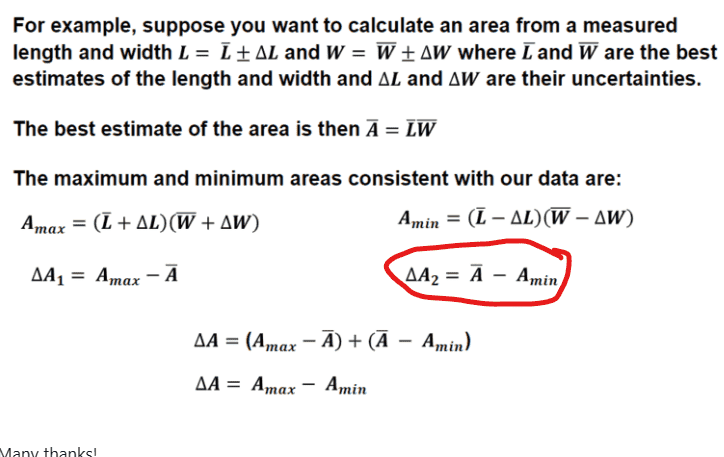# Uncertainties propagation for area of a rectangle

• ChiralSuperfields

#### ChiralSuperfields

Homework Statement
I am trying to understand how they got the line ##\Delta A_2 = \bar A - A_{min}## below

When I expand the expression above it ##A_{min} = (\bar L - \Delta L)(\bar W - \Delta W) ## I get ##A_{min} = \bar L \bar W - \Delta W \bar L - \Delta L \bar W + \Delta L \Delta W = \bar A + \Delta A_2##
Relevant Equations
Pls see belowMany thanks!

#### Attachments

Homework Statement:: I am trying to understand how they got the line ##\Delta A_2 = \bar A - A_{min}## below

When I expand the expression above it ##A_{min} = (\bar L - \Delta L)(\bar W - \Delta W) ## I get ##A_{min} = \bar L \bar W - \Delta W \bar L - \Delta L \bar W + \Delta L \Delta W = \bar A + \Delta A_2##
They didn't "get it." ##\Delta A_2=\bar A-A_{min}## is a definition just like ##\Delta A_1.## They are the differences from the mean value to the high value ##A_{max}## and the the low value ##A_{min}##. The assertion is that the overall uncertainty is the the distance from the average value to the high value plus the distance from the average value to the low value. This is a simplified way to treat uncertainties.

•topsquark and ChiralSuperfields
They didn't "get it." ##\Delta A_2=\bar A-A_{min}## is a definition just like ##\Delta A_1.## They are the differences from the mean value to the high value ##A_{max}## and the the low value ##A_{min}##. The assertion is that the overall uncertainty is the the distance from the average value to the high value plus the distance from the average value to the low value. This is a simplified way to treat uncertainties.

That is very helpful that you mention that ##\Delta A_1## and ##\Delta A_2## are both definitions. Before, I thought I had derived ##A_{max} = \bar A + \Delta A_2## from ##A_{max} = \bar L \bar W + \Delta W \bar L + \Delta L \bar W + \Delta L \Delta W = \bar A + \Delta A_2##

Are why not allowed to compare the two expressions and say that ##\Delta A_2 = \Delta W \bar L + \Delta L \bar W + \Delta L \Delta W##?

Many thanks!

You are allowed to compare anything you want. What is actually your question about this? I already explained what they are trying to do but maybe you didn't understand it. I will say it differently.
You want to find an area. To do this, you make many measurements of the length ##L## and the width ##W##. These measurements are not identical so you have uncertainties ##\Delta L## and ##\Delta W##.

Now you can calculate average values ##\bar L## and ##\bar W## and use these to find an average for the area ##\bar A= \bar L\bar W.## You also need to calculate the uncertainty ##\Delta A## in the value for the area. The insert that you posted shows you how to do this. It is the distance (in area units) between the maximum value and the minimum value for the area. So I will ask you again, what specifically is it about this that you don't understand?

Last edited:
•topsquark and ChiralSuperfields
You are allowed to compare anything you want. What is actually your question about this? I already explained what they are trying to do but maybe you didn't understand it. I will say it differently.
You want to find an area. To do this, you make many measurements of the length ##L## and the width ##W##. These measurements are not identical so you have uncertainties ##\Delta L## and ##\Delta W##.

Now you can calculate average values ##\bar L## and ##\bar W## and use these to find an average for the area ##\bar A= \bar L\bar W.## You also need to calculate the uncertainty ##\Delta A## in the value for the area. The insert that you posted shows you how to do this. It is the distance (in area units) between the maximum value and the minimum value for the area. So I will ask you again, what specifically is it about this that you don't understand?
Thank you for your help @kuruman!

I think I understand now :)

If I don't I will come back to this thread

Are why not allowed to compare the two expressions and say that ##\Delta A_2 = \Delta W \bar L + \Delta L \bar W + \Delta L \Delta W##?
You are allowed to compare two expressions, but only if the result is correct.

As was already stated, ##A_2 = \bar A - A_{min} = (\bar L - \Delta L)(\bar W - \Delta W)##
## = \bar L \bar W - \Delta L \bar W - \Delta W \bar L + \Delta L \Delta W##
What you wrote omits the ##\Delta W \bar L## term, which is equal to ##\bar A##.

Last edited:
•ChiralSuperfields